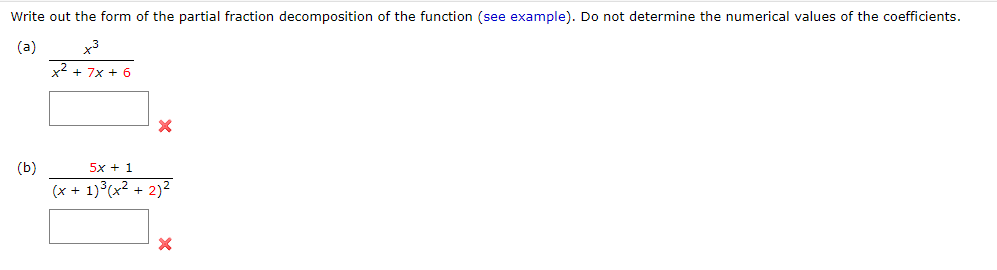Home / Expert Answers / Calculus / write-out-the-form-of-the-partial-fraction-decomposition-of-the-function-see-example-do-not-det-pa853

# (Solved): Write out the form of the partial fraction decomposition of the function (see example). Do not det ...Write out the form of the partial fraction decomposition of the function (see example). Do not determine the numerical values of the coefficients. (a) (b)

We have an Answer from Expert